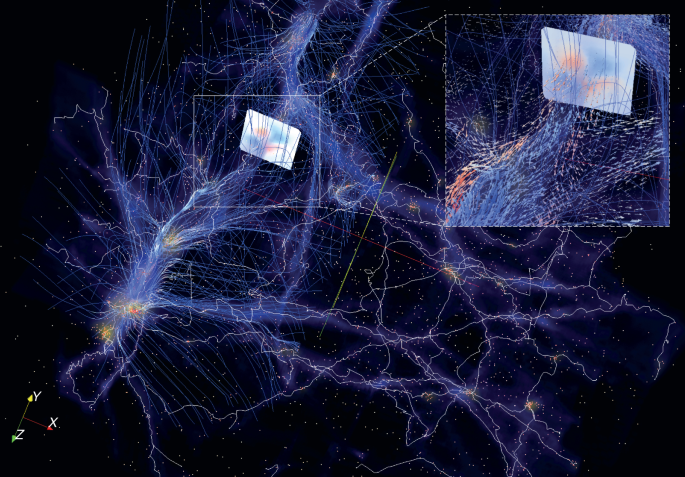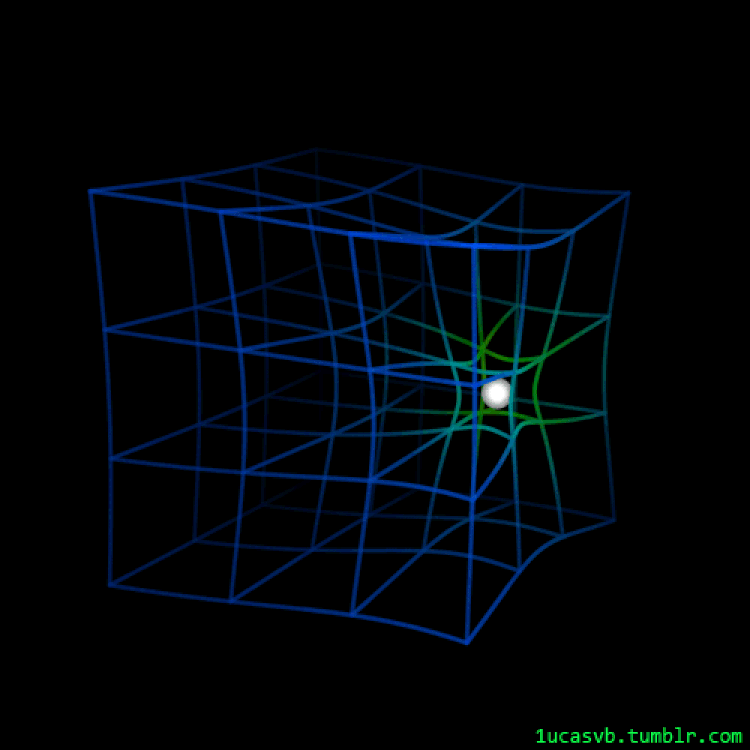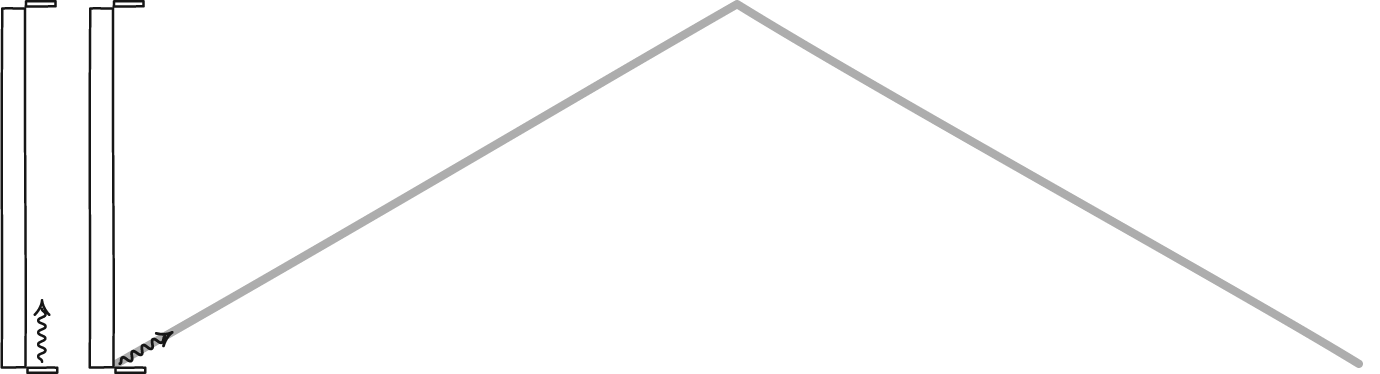If there’s one equation that people learn about physics — and no, not Einstein’s E = mc2 — it’s Newton’s F = ma. Despite the fact that it’s been in widespread use for some ~350 years now, since Newton first put it forth in the late 17th century, it rarely makes the list of most important equations. Yet it’s the one that physics students learn more than any other at the introductory level, and it remains important as we advance: through our undergraduate educations, through graduate school, in both physics and engineering, and even when we move on to engineering, calculus, and some very intense and advance concepts.

F = ma, despite its apparent simplicity, keeps on delivering new insights to those who study it, and has done so for centuries. Part of the reason for why it’s so undervalued is because it’s so ubiquitous: after all, if you’re going to learn anything about physics, you’re going to learn about Newton, and this very equation is the key statement of Newton’s 2nd law. In addition, it’s just three parameters — force, mass, and acceleration — related through an equals sign. While it might seem like there’s very little to it, the truth is that there’s a fantastic world of physics that opens up if you only investigate the depths of F = ma. Let’s dive in.In isolation, any system, whether at rest or in motion, including angular motion, will be unable to change that motion without an outside force. In space, your options are limited, but even in the International Space Station, one component (like an astronaut) can push against another (like another astronaut) to change the individual component’s motion: the hallmark of Newton’s laws in all their incarnations. (Credit: NASA/International Space Station)

## The Basics

The first time you get an equation like F = ma, it’s simple to treat it the same way you’d treat an equation for a line in mathematics. Additionally, it seems like it’s even a little bit simpler: instead of an equation like y = mx + b, for instance, which is the classic math formula for a line, there’s no “b” in there at all.

Why is that?

Because this is physics, not mathematics. We only write down equations that are physically consistent with the Universe, and any b that isn’t zero would lead to pathological behavior in physics. Remember that Newton put forth three laws of motion describing all bodies:

1. An object at rest remains at rest and an object in motion remains in constant motion, unless acted upon by an outside force.
2. An object will accelerate in the direction of whatever net force is applied to it, and will accelerate with a magnitude of that force divided by the object’s mass.
3. Any action — and a force is an example of an action — must have an equal and opposite reaction. If anything exerts a force on any object, that object exerts an equal and opposite force on the “thing” that pushes or pulls it.

The first law is the reason that the equation is F = ma and not F = ma + b, because otherwise objects could not remain in constant motion in the absence of outside forces.An object at rest will remain at rest, unless acted upon by an outside force. As a result of that outside force, the coffee cup is no longer at rest. (Credit: gfpeck/flickr)

This equation, then, F = ma, has three meanings associated with it, at least in a physical sense and without further unpacking of what a force, a mass, or an acceleration means.

• If you can measure the mass of your object and how it’s accelerating, you can use F = ma to determine the net force acting on the object.
• If you can measure the mass of your object and you know (or can measure) the net force being applied to it, you can determine how that object will accelerate. (This is particularly useful when wanting to determine how an object will accelerate under the influence of gravity.)
• And if you can measure or know both the net force on an object and how it’s accelerating, you can use that information to determine your object’s mass.

Any equation with three variables connected like this, where one variable is on one side of the equation and the other two are multiplied together on the other side, behaves exactly like this. Other famous examples include Hubble’s law for the expanding Universe, which is v = Hr (the recession speed equals the Hubble constant multiplied by distance), and Ohm’s Law, which is V = IR (voltage equals current multiplied by resistance).

We can think of F = ma in two other ways that are equivalent: F/m = a and F/a = m. Although it’s only algebraic manipulation to get these other equations from the original, it’s a useful exercise in teaching introductory students to solve for an unknown quantity using the physical relationships and the known quantities we do possess.In this stop-motion composite, a man starts at rest and accelerates by exerting a force between his feet and the ground. If two of the three of force, mass, and acceleration are known, you can find the missing quantity through properly applying Newton’s F = ma. (Credit: rmathews100/Pixabay)

The way to take F = ma to the next level is simple and straightforward, but also profound: it’s to realize what acceleration means. An acceleration is a change in velocity (v) over a time (t) interval, and this can either be an average acceleration, such as taking your car from 0 to 60 mph (approximately the same as going from 0 to 100 kph), or an instantaneous acceleration, which asks about your acceleration at one particular moment in time. We normally express this as a = Δv/Δt, where the “Δ” symbol stands for a change between a final and an initial value, or as a = dv/dt, where the “d” denotes an instantaneous change.

Similarly, velocity itself is a change in position (x) over time, so we can write v = Δx/Δt for an average velocity, and v = dx/dt for an instantaneous velocity. The relationship between position, velocity, acceleration, force, mass, and time is a profound one: one that scientists puzzled about for decades, generations, and even centuries before the very basic equations of motions were successfully written down in the 17th century.

In addition, you’ll notice that some of the letters are bolded: x, v, a, and F. That’s because they’re not just quantities; they’re quantities with directions associated with them. Given that we live in a three-dimensional Universe, every one of these equations with a “bold” quantity in it is actually three equations: one for each of the three dimensions (e.g., x, y, and z directions) present in our Universe.The fact that F = ma is a three-dimensional equation doesn’t always lead to complications arising between dimensions. Here, a ball under the influence of gravity accelerates only in the vertical direction; its horizontal motion remains constant, so long as air resistance and energy loss from impacting the ground are neglected. (Credit: MichaelMaggs Edit by Richard Bartz/Wikimedia Commons)

One of the remarkable things about these sets of equations is this: they’re all independent of one another.

What happens in the x-direction, in terms of force, position, velocity, and acceleration, only affects the other components in the x-direction. Similarly for the y-and-z-directions as well: what happens in those directions only affects those directions. It’s why when you hit a golf ball on the Moon, gravity only affects its motion in the “up-and-down” direction, not in the side-to-side direction; it will continue on, constantly, with its motion unchanged: it’s an object in motion with no external forces in that direction.

We can extend this motion in a number of powerful ways. Instead of treating objects as though they’re idealized point masses, we can consider masses that are extended objects. Instead of treating objects that only move in lines, accelerating at a constant rate in one or more directions, we can treat objects that orbit and rotate. Through this procedure, we can begin to discuss concepts like torque, moment of inertia, as well as angular position, angular velocity, and angular acceleration. Newton’s laws and equations of motion all still apply here, as everything in this discussion can be derived from that same core equation: F = ma.The fact that structures in the Universe exert forces on one another as they move, and that these structures are extended objects rather than point sources, can lead to torques, angular accelerations, and rotational motions. The application of F = ma to complex systems is enough, on its own, to account for this. (Credit: K. Kraljic, Nature Astronomy, 2021)

## Calculus and Rates

There’s an important physical reality that we’ve been dancing around, but it’s time to take it on directly: the concept of a rate. Velocity is the rate at which your position changes: it’s a distance over a time, or a change in distance over a change in time, and that’s why it has units like meters-per-second or miles-per-hour. Similarly, acceleration is the rate at which your velocity changes: it’s a change in velocity over a change in time, and that’s why it has units like meters-per-second2: because it’s a velocity (meters-per-second) over a time (per-second).

If you know, for example:

• where something is right now,
• what time it is right now,
• how quickly it’s moving right now,
• and what forces are and will be acting on it,

you can predict what it will do in the future. That means we can predict where it will be at any point in time, including arbitrarily far into the future, so long as we have sufficient computational or calculational power at our disposal. Newton’s equations are entirely deterministic, and so if we can measure or know what an object’s initial conditions are at some time, and we know how that object will experience forces over time, we can predict precisely where it will wind up.While planetary motion may look simple, it’s governeed by a second-order differential equation relating force to acceleration. The difficulty in solving this equation should not be underestimated, but the power of Newton’s F = ma in explaining an enormous variety of phenomena in the Universe shouldn’t be underestimated either. (Credit: J. Wang (UC Berkeley) & C. Marois (Herzberg Astrophysics), NExSS (NASA), Keck Obs.)

This is how we predict planetary motion, comet arrivals, assess asteroids for their potential to strike Earth, and how we made it to the Moon. At its core, F = ma is what we call a differential equation, and a second-order differential equation at that. (Why? Because “second-order” means it has a second time-derivative in there: acceleration is a change in velocity over a change in time, while velocity is a change in position over a change in time.) Differential equations are their own branch of mathematics, and the best descriptions I know of them are twofold.

• A differential equation is an equation that tells you, assuming you know what your object is doing right now, what it will be doing at the very next moment. Then, when that next moment has elapsed, that very same equation tells you what will occur at the subsequent moment, and so on, forward to infinity.
• However, most of the differential equations that exist cannot be exactly solved; we can only approximate them. Furthermore, most of the differential equations that can be solved cannot be solved by us, and by “us” I mean professional theoretical physicists and mathematicians. These things are hard.

F = ma is one of those very hard differential equations, and yet the comparatively simple circumstances under which we can solve it are incredibly educational. This fact underlies much of the work that we’ve done in theoretical physics for centuries, a fact that remains true even today.An animated look at how spacetime responds as a mass moves through it helps showcase exactly how, qualitatively, it isn’t merely a sheet of fabric but all of space itself gets curved by the presence and properties of the matter and energy within the Universe. Note that spacetime can only be described if we include not only the position of the massive object, but where that mass is located throughout time. Both instantaneous location and the past history of where that object was located determine the forces experienced by objects moving through the Universe, making General Relativity’s set of differential equations even more complicated than Newton’s. (Credit: LucasVB)

## It leads us to Rockets and Relativity

This is one of those, “huh, what?” moments for most people when they learn about it. It turns out all this time, physics teachers have been telling you a little white lie about F = ma.

The lie?

Newton himself never wrote it or formulated it like this in any way. He never said, “force equals mass times acceleration.” Instead, he said, “force is the time rate of change of momentum,” where momentum is the product of mass times velocity.

These two statements are not the same. F = ma tells you that force, which occurs in some direction, leads to an acceleration of masses: a changing velocity over time for every mass that experiences a force. Momentum, which physicists unintuitively (for English speakers) represent with the letter p, is the product of mass times velocity: p = mv.

Can you see the difference? If we change momentum over time, whether it’s with average momentum (Δp/Δt) or with instantaneous momentum (dp/dt), we run into an issue. Writing down F = ma makes the assumption that mass does not change; only velocity changes. This isn’t universally true, however, and the two big exceptions have been hallmarks of 20th century advances.This photograph shows the 2018 launch of Rocket Lab’s Electron rocket lifting off from Launch Complex 1 in New Zealand. Rockets convert fuel into energy and thrust, expelling it and losing mass as they accelerate. As a result, F = ma is too oversimplified to be used to calculate a rocket’s acceleration. (Credit: Trevor Mahlmann/Rocket Lab)

One is the science of rocketry, since rockets actively lose their mass (burning it and expelling it as exhaust) as they actively accelerate. In fact, the “changing mass, also” version of the equation, where both velocity and mass are allowed to vary over time, is known by many as simply “the rocket equation.” When a loss or gain in mass is occurring, it affects your objects motion, and how that motion changes over time as well. Without the mathematics of calculus and differential equations, and without the physics of how objects such as this behave in real life, calculating the behavior of a spacecraft powered by propellant would be impossible.

The other is the science of special relativity, which becomes important when objects move close to the speed of light. If you use Newton’s equations of motion, and the equation F = ma to calculate how an object’s position and speed change when you apply a force to it, you can incorrectly calculate conditions that lead to your object exceeding the speed of light. If, however, you instead use F = m(dp/dt) as your force law — the way Newton himself wrote it — then so long as you remember to use relativistic momentum (where you add in a factor of the relativistic γ: p = v), you’ll find that the laws of special relativity, including time dilation and length contraction, all naturally appear.This illustration of a light-clock shows how, when you’re at rest (left), a photon travels up-and-down between two mirrors at the speed of light. When you’re boosted (moving to the right), the photon also moves at the speed of light, but takes longer to oscillate between the bottom and the top mirror. As a result, time is dilated for objects in relative motion compared to stationary ones. (Credit: John D. Norton/University of Pittsburgh)

Many have speculated, based on this observation and the fact that Newton could have easily written F = ma instead of F = m(dp/dt), that perhaps Newton actually anticipated special relativity: an assertion with no way to disprove it. However, regardless of what was going on in Newton’s head, it’s undeniable that there’s a tremendous rabbit-hole of insight into the workings of our Universe — along with the development of invaluable tools for problem solving — embedded in the simple-looking equation behind Newton’s second law: F = ma.

Every time a particle moves through curved spacetime; every time an object experiences a push, pull, or forceful interaction with another entity; every time a system does anything other than remain at rest or in constant, unchanging motion, the idea of forces and accelerations will come into play. Even though Newton’s F = ma isn’t universally true in all circumstances, its tremendous range of validity, the deep physical insights that it holds, and the interrelationships it encodes across systems both simple and complex ensures its status as one of the most important equations in all of physics. If you’re only going to teach one physics equation to someone, make it this one. With enough effort, you can use it to decode the workings of almost the entire Universe.